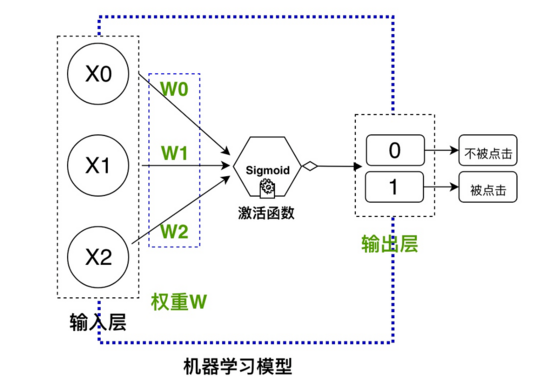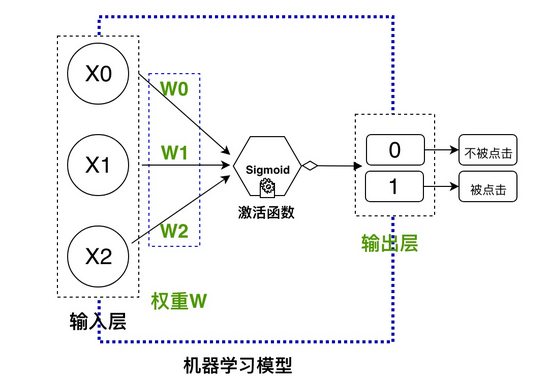# 从零开始学习神经网络：一个快速入门Python机器学习的经典实战项目0.前言

1.假设一个业务场景

X={x0="该微博有娱乐明星”，x1="该微博有图”，x2="该微博有表情”}

Y={y0=“点击”, y1=“未点击”}

2.任务分析：

3.数据准备：4.神经网络基本结构：

1.输入层：输入的业务特征数据

2.隐藏层：初始化权重参数

3.激活函数：选择激活函数

4.输出层：预测的目标，定义损失函数5.使用Python代码构建网络

 12345678910111213141516171819202122232425262728293031323334353637383940414243444546474849505152535455565758596061626364656667 # coding:utf-8import numpy as np class NeuralNetwork(): �# 随机初始化权重�def __init__(self): ��np.random.seed(1) ��self.synaptic_weights = 2 * np.random.random((3, 1)) - 1�# 定义激活函数：这里使用sigmoid�def sigmoid(self, x): ��return 1 / (1np.exp(-x)) �#计算Sigmoid函数的偏导数 �def sigmoid_derivative(self, x): ��return x * (1 - x)�# 训练模型 �def train(self, training_inputs, training_outputs,learn_rate, training_iterations): ��# 迭代训练��for iteration in range(training_iterations): ���#前向计算 ���output = self.think(training_inputs) ���# 计算误差 ���error = training_outputs - output ���# 反向传播-BP-微调权重 ���adjustments = np.dot(training_inputs.T, error * self.sigmoid_derivative(output)) ���self.synaptic_weights = learn_rate*adjustments �def think(self, inputs): ��# 输入通过网络得到输出 ��# 转化为浮点型数据类型 ��inputs = inputs.astype(float) ��output = self.sigmoid(np.dot(inputs, self.synaptic_weights)) ��return output if __name__ == "__main__": �# 初始化前馈神经网络类 �neural_network = NeuralNetwork() �print "随机初始化的权重矩阵W"�print neural_network.synaptic_weights�# 模拟训练数据X�train_data=[[0,0,1], [1,1,1], [1,0,1], [0,1,1]]�training_inputs = np.array(train_data) �# 模拟训练数据Y�training_outputs = np.array([[0,1,1,0]]).T �# 定义模型的参数：�# 参数学习率�learn_rate=0.1�# 模型迭代的次数�epoch=150000�neural_network.train(training_inputs, training_outputs, learn_rate, epoch) �print "迭代计算之后权重矩阵W: "�print neural_network.synaptic_weights�# 模拟需要预测的数据X�pre_data=[0,0,1]�# 使用训练的模型预测该微博被点击的概率�print "该微博被点击的概率："�print neural_network.think(np.array(pre_data))"""终端输出的结果：随机初始化的权重矩阵W[[-0.16595599]�[ 0.44064899]�[-0.99977125]]迭代计算之后权重矩阵W: [[12.41691302]�[-0.20410552]�[-6.00463275]]该微博被点击的概率：[0.00246122][Finished in 20.2s]"""

6.总结：